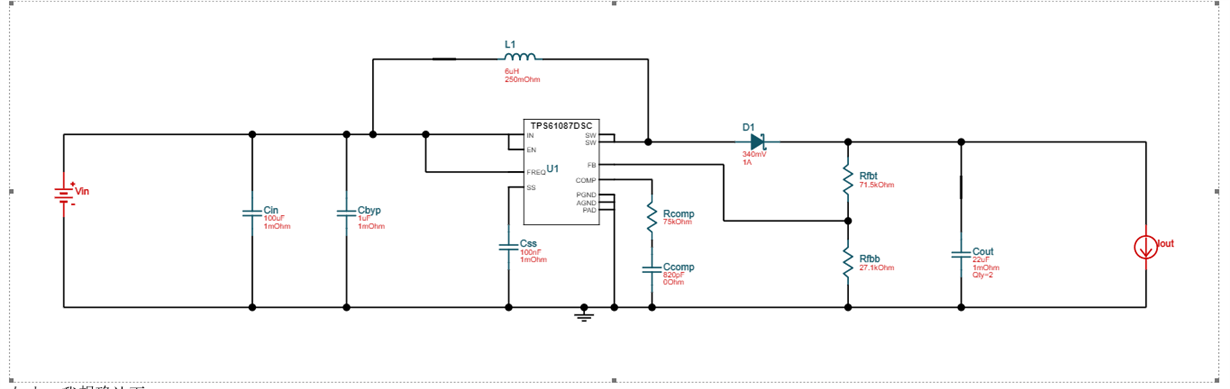If you have a related question, please click the "Ask a related question" button in the top right corner. The newly created question will be automatically linked to this question.

• TI Thinks Resolved

# TPS61087: inductor question fpr TPS61087

Part Number: TPS61087

Hi team,

I am a hardware engineer. Now I will use TPS61087.  Input=3.3V, Output:4.5V, The max output current is 100mA.

Q1: I want to confirm my circuit works in CCM mode or DCM mode?

The datasheet shows"The converter operates in continuous conduction mode (CCM) as soon as the input current increases above half the ripple current in the inductor, for lower load currents it switches into

discontinuous conduction mode (DCM). If the load is further reduced, the part starts to skip pulses to maintain the output voltage."  So, I guess my circuit work in DCM mode, correct?

Q2: If my circuit work in DCM mode, Can I use the formula in 8.2.2 Detailed Design Procedure of the datasheetDoes the formula only be used for CCM mode?

Q3: Also, I use the 8.2.2 Detailed Design Procedure formula to calculate the inductor peak current. The inductor peak is  about 360mA When I choose 2.2uH inductor, Fs=1.2M.

If the 8.2.2 Detailed Design Procedure formula can be suitable for my circuit, I will select the  inductor saturation current is 1.7A.

When I use 8.2.2.1 Inductor Selection formula to calculate the inductor value. VIN=3.3V, VS=4.5V, IOUT=100mA, FS=1.2M, η=90%, L is about 13uH. It is very large.

And , Wehn I use Webwench, The circuit is as below:The inductor is 6uH.

I want to verify why the inductor value that is 8.2.2.1 Inductor Selection formula  is very big?

I want to use the smaller inductor package for my application since my board size is limited, such as 2.2uH.

So, How can I select the inductor?

Q4: Converter switching frequency:

When FREQ pin is connected to VIN, the typ switching frequency is 1.2M.

When my output current is 100mA or less than 100mA that is very LOW courrent,  will  the switching frequency be dropped or keep 1.2M?

I note  the switching frequency will be dropped when the load current is low in some boost devices. Because the boost

device will enter the burst mode when the load current is very low.

• Dear Sir,

You can simulate by Webench to determine it works in the DCM or CCM. For the TPS61087, if the load current is very small, then it will enter into the burst mode.

For the inductor selection, if the load current is small, like in your application, the load current is only 100mA, then you you can select a smaller inductor, a 3.3-4.7uH inductor is also OK. Because in the high current application, the inductor ripple current ratio can be 0.3, but in the low current application, the inductor ripple can be 0.5-1.0 or even bigger.

You can change the inductor value to check the result in the webench.

Best Regards,
Helen

Boost DC/DC Solution